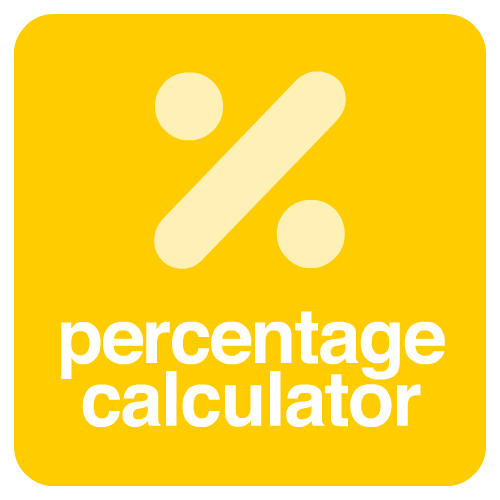Aspect Ratios

# percentage calculator

What is
% of
=
is how many percent of
=

### %

What is the percentage increase / decrease
From
to
=

### %

Adds or subtracts percentage from a number
=## The most complete percentage calculator!

Percentage calculator is a powerful tool for calculating any type of percentage, such as increase or decrease percentage. You no longer need to worry when you need to calculate percentage. Use our calculator simply and quickly, and the best, completely free!

### Discover all the features of our calculator!

There are several ways to calculate a percentage, and our tool does it with ease. If you need to know the variation from one value to another or calculate, for example, or how many percent a value will increase. Whatever accounts you need to use, use our calculator and save time. But if you still have any questions, see our explanations below.

### tips and information

#### Percentage calculator of a number

When you need to know how much is a percentage over a value, for example how much is 20% of 300. In this case the result is 60

#### Percentage of one number in relation to another

When you need to know how many percent one number is in relation to the other. For example, if you need to know how many percent 100 is in relation to 200. In this case 100 is 50% of 200

#### Percentage variation between two numbers

In this case you can use it if you need to know the percentage of variation between two numbers. For example, if you need to know how many percent is the variation between the original number and the final number. If your original number is 100 and your final number is 70, the variation is -30%

#### Add percentage to a number

When you want to increase or decrease a percentage to a number. For example, if you have 1000 and want to add 75%, in this case the final number results in 1750